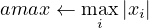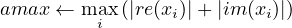#### 4.4 psb_normi — Infinity-Norm of Vector

This function computes the infinity-norm of a vector x.
If x is a real vector it computes infinity norm as:else if x is a complex vector then it computes the infinity-norm as:psb_geamax(x, desc_a, info [,global])
psb_normi(x, desc_a, info [,global])

 amax x Function Short Precision Real Short Precision Real psb_geamax Long Precision Real Long Precision Real psb_geamax Short Precision Real Short Precision Complex psb_geamax Long Precision Real Long Precision Complex psb_geamax

Table 4: Data types

Type:
Synchronous.
On Entry
x
the local portion of global dense matrix x.
Scope: local
Type: required
Intent: in.
Specified as: a rank one or two array or an object of type psb_T_vect_type containing numbers of type specified in Table 4.
desc_a
contains data structures for communications.
Scope: local
Type: required
Intent: in.
Specified as: an object of type psb_desc_type.
global
Specifies whether the computation should include the global reduction across all processes.
Scope: global
Type: optional.
Intent: in.
Specified as: a logical scalar. Default: global=.true.
On Return
Function value
is the infinity norm of vector x.
Scope: global unless the optional variable global=.false. has been specified
Specified as: a long precision real number.
info
Error code.
Scope: local
Type: required
Intent: out.
An integer value; 0 means no error has been detected.

Notes

1. The computation of a global result requires a global communication, which entails a significant overhead. It may be necessary and/or advisable to compute multiple norms at the same time; in this case, it is possible to improve the runtime efficiency by using the following scheme:
vres(1) = psb_geamax(x1,desc_a,info,global=.false.)
vres(2) = psb_geamax(x2,desc_a,info,global=.false.)
vres(3) = psb_geamax(x3,desc_a,info,global=.false.)
call psb_amx(ctxt,vres(1:3))

In this way the global communication, which for small sizes is a latency-bound operation, is invoked only once.